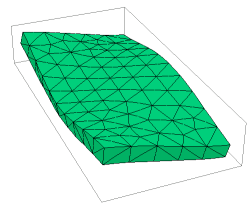﻿ Sample Problems > Usage > Eigenvalues > 3d_plate

# 3d_plate

Navigation:  Sample Problems > Usage > Eigenvalues >

# 3d_plate{ 3D_PLATE.PDE

This problem considers the oscillation modes of a glass plate in space

( no mountings to constrain motion ).

-- Submitted by John Trenholme, Lawrence Livermore Nat'l Lab.

}

 TITLE 'Oscillation of a Glass Plate'   COORDINATES  cartesian3   SELECT    modes = 5    ngrid=10    errlim = 0.01 { 1 percent is good enough }   VARIABLES    U           { X displacement }    V           { Y displacement }    W           { Z displacement }   DEFINITIONS    cm = 0.01       { converts centimeters to meters }      long = 20*cm   { length of plate along Y axis }    wide = 10*cm   { width of plate along X axis }    thick = 1.2*cm { thickness of plate along Z axis }      E = 50e9       { Youngs modulus in Pascals }    nu = 0.256     { Poisson's ratio  }    rho = 2500     { density in kg/m^3 = 1000*[g/cc] }     { constitutive relations - isotropic material }    G = E/((1+nu)*(1-2*nu))    C11 = G*(1-nu)    C12 = G*nu    C13 = G*nu    C22 = G*(1-nu)    C23 = G*nu    C33 = G*(1-nu)    C44 = G*(1-2*nu)/2{ Strains }

ex = dx(U)    ey = dy(V)    ez = dz(W)

gxy = dy(U) + dx(V)    gyz = dz(V) + dy(W)    gzx = dx(W) + dz(U)

{ Stresses }

Sx  =  C11*ex + C12*ey + C13*ez

Sy  =  C12*ex + C22*ey + C23*ez

Sz  =  C13*ex + C23*ey + C33*ez

Txy =  C44*gxy    Tyz =  C44*gyz    Tzx =  C44*gzx

{ find mean Y and Z translation and X rotation }

Vol = Integral(1)

{ scaling factor for displacement plots }

Mt =0.1*globalmax(magnitude(x,y,z))/globalmax(magnitude(U,V,W))

INITIAL VALUES

U = 1.0e-5    V = 1.0e-5    W = 1.0e-5

EQUATIONS

{ we assume sinusoidal oscillation at angular frequency omega =sqrt(lambda) }

U:  dx(Sx) + dy(Txy) + dz(Tzx) + lambda*rho*U = 0   { X-displacement equation }

V:  dx(Txy) + dy(Sy) + dz(Tyz) + lambda*rho*V = 0   { Y-displacement equation }

W:  dx(Tzx) + dy(Tyz) + dz(Sz) + lambda*rho*W = 0   { Z-displacement equation }

CONSTRAINTS

integral(U)=0               { eliminate translations }

integral(V)=0

integral(W)=0

integral(dx(V)-dy(U)) = 0   { eliminate rotations }

integral(dy(W) - dz(V)) = 0

integral(dz(U) - dx(W))  = 0

EXTRUSION

surface "bottom" z = -thick / 2

layer "plate"

surface "top" z = thick / 2

BOUNDARIES

region 1 { all sides, and top and bottom, are free }

start( -wide/2, -long/2 )

line to ( wide/2, -long/2 )

line to ( wide/2, long/2 )

line to ( -wide/2, long/2 )

line to close

MONITORS

grid(x+Mt*U,y+Mt*V,z+Mt*W) as "Shape"

report sqrt(lambda)/(2*pi) as "Frequency in Hz"

PLOTS

contour( W ) on z = 0 as "Mid-plane Displacement"

report sqrt(lambda)/(2*pi) as "Frequency in Hz"

grid(x+Mt*U,y+Mt*V,z+Mt*W) as "Shape"

report sqrt(lambda)/(2*pi) as "Frequency in Hz"

summary

report lambda

report sqrt(lambda)/(2*pi) as "Frequency in Hz"

END